# Lab 10. SNMP Batch File Exercise 1 Write a batch file to meet the following requirements...

Lab 10. SNMP Batch File

Exercise 1

Write a batch file to meet the following requirements

Name the file: batch1.bat

Use loop technique to retrieve the Agent’s OID values ipInReceives, ipOutRequests and ipOutDiscards every 5 seconds for 3 times

.

Display the result (timestamps and OID values) on the Command Shell window and write it to: batch1_result.tx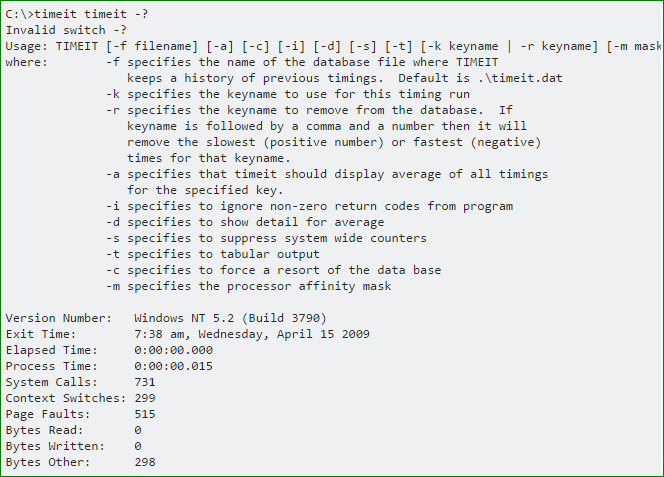#### Earn Coin

Coins can be redeemed for fabulous gifts.

Similar Homework Help Questions
• ### Windows operating system 1. Write a simple batch file named LastName Final.bat (where LastName is your...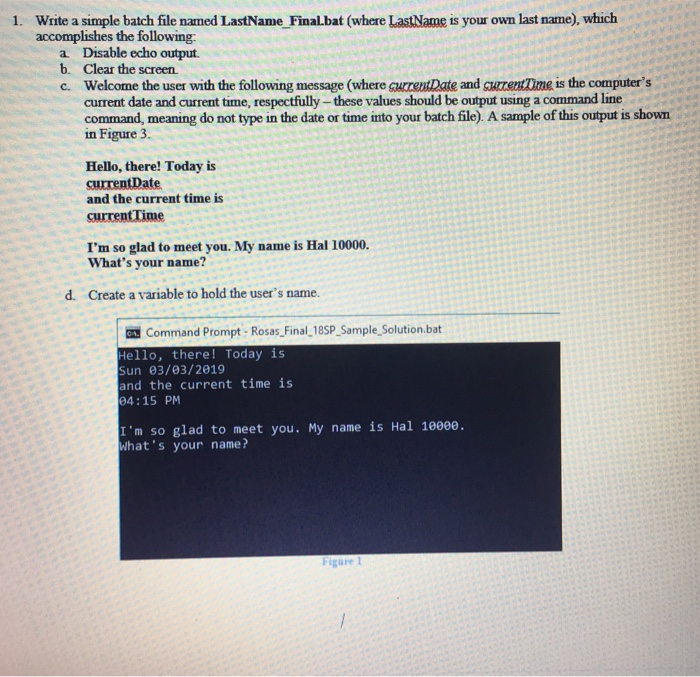Windows operating system 1. Write a simple batch file named LastName Final.bat (where LastName is your own last name), which accomplishes the following: a Disable echo output b. Clear the screen c. Welcome the user with the following message (where current Date and curent Time is the computer's current date and current time, respectfully-these values should be output using a command line command, meaning do not type in the date or time into your batch file). A sample of this...

• ### Student ID: 123 Write a C+ program with the following specifications: a. Define a C++ function (name it function_Student...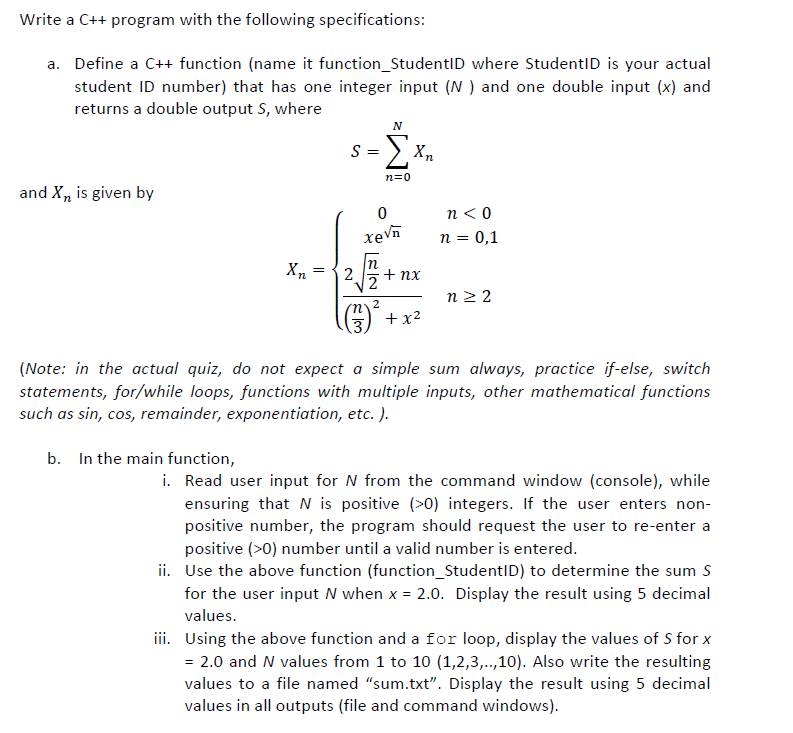Student ID: 123 Write a C+ program with the following specifications: a. Define a C++ function (name it function_StudentlD where StudentID is your actual student ID number) that has one integer input (N) and one double input (x) and returns a double output S, where N S = n 0 and X2 is given by 0 xeVn n 0,1 Хл —{2. nx 2 n 2 2 m2 x2 3 (Note: in the actual quiz, do not expect a always, practice...

• ### Use C++ For this week’s lab you will write a program to read a data file...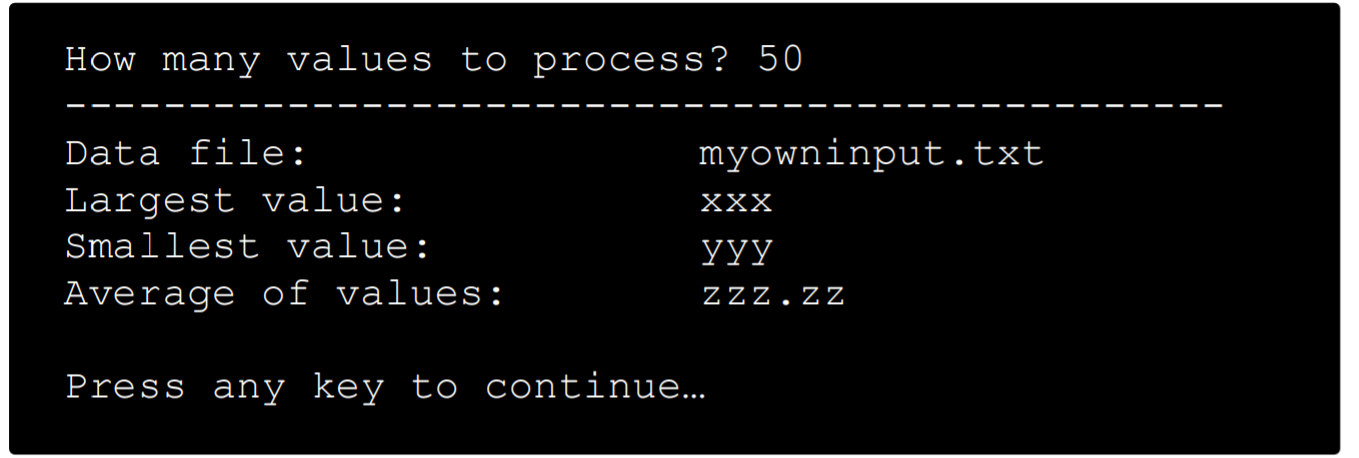Use C++ For this week’s lab you will write a program to read a data file containing numerical values, one per line. The program should compute average of the numbers and also find the smallest and the largest value in the file. You may assume that the data file will have EXACTLY 100 integer values in it. Process all the values out of the data file. Show the average, smallest, largest, and the name of the data file in the...

• ### Function / File Read (USING MATLAB) A) create a user-defined function : [maxsample , maxvalue , numsamples]=writetofile(sname,strgth) to do the following: The user-defined function shall: -Accept as t...

Function / File Read (USING MATLAB) A) create a user-defined function : [maxsample , maxvalue , numsamples]=writetofile(sname,strgth) to do the following: The user-defined function shall: -Accept as the input a provided string for the sample name (sname) and number for strength (strgth) - open a text file named mytensiledata.txt, with permission to read or append the file. - Make sure to capture any error in opening the file - Write sname and strgth to the file - Close the file...

• ### Instructions: For your lab write-up follow the instructions of LAB 1 1. (a) Modify the function e...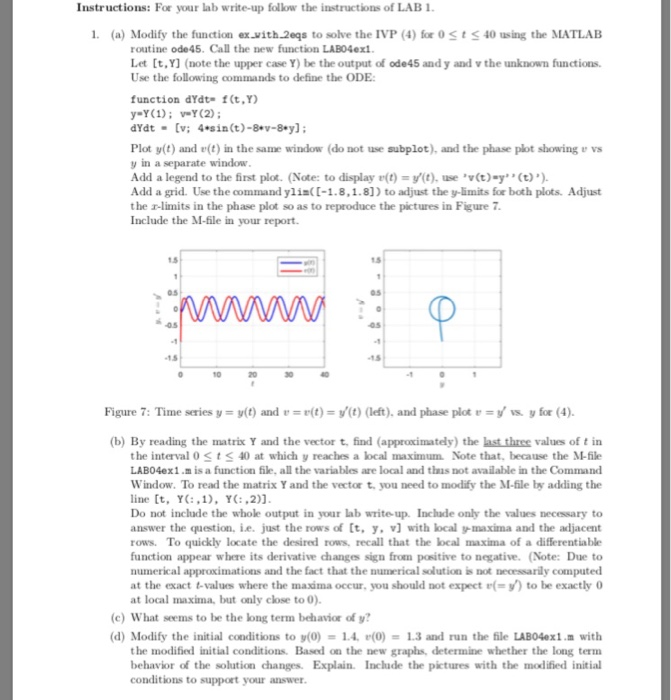Instructions: For your lab write-up follow the instructions of LAB 1 1. (a) Modify the function exvith2eqs to solve the IVP (4) for o St S40 using the MATLAB routine ode45. Call the new function LAB04exl Let [t,Y] (note the upper case Y) be the output of ode45 and y and v the unknown functions. Use the following commands to define the ODE function dYdt f(t.Y) y-Y();Y(2) Plot y(t) and e(t) in the same window (do not use subplot), and...

• ### Methods and File Output and Exceptions Outcome: Student will demonstrate the ability to write, use and...

Methods and File Output and Exceptions Outcome: Student will demonstrate the ability to write, use and call methods Student will demonstrate the ability to pass values to and from methods Student will demonstrate the ability to catch exceptions Student will demonstrate the ability to create a text file Student will demonstrate the ability to validate input data Program Specifications: You to write a menu driven program. The program will use a switch statement. The cases will be as follows: Get...

• ### use MatLab to answer these questions 1. (10 points) Create an m-file called addup.m Use a...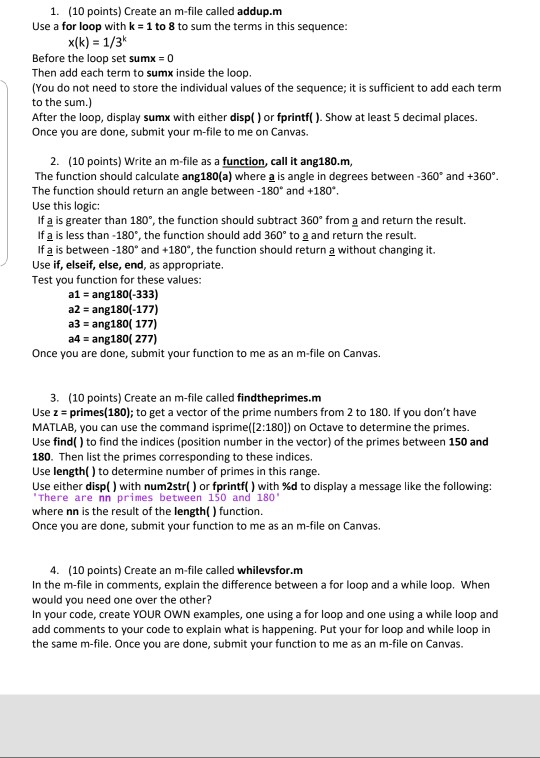use MatLab to answer these questions 1. (10 points) Create an m-file called addup.m Use a for loop with k = 1 to 8 to sum the terms in this sequence: x(k) = 1/3 Before the loop set sumx = 0 Then add each term to sumx inside the loop. (You do not need to store the individual values of the sequence; it is sufficient to add each term to the sum.) After the loop, display sumx with either disp()...

• ### ( Object array + input) Write a Java program to meet the following requirements: 1. Define...

( Object array + input) Write a Java program to meet the following requirements: 1. Define a class called Student which contains: 1.1 data fields: a. An integer data field contains student id b. Two String data fields named firstname and lastname c. A String data field contains student’s email address 1.2 methods: a. A no-arg constructor that will create a default student object. b. A constructor that creates a student with the specified student id, firstname, lastname and email_address...

• ### (Java) Rewrite the following exercise below to read inputs from a file and write the output...

(Java) Rewrite the following exercise below to read inputs from a file and write the output of your program in a text file. Ask the user to enter the input filename. Use try-catch when reading the file. Ask the user to enter a text file name to write the output in it. You may use the try-with-resources syntax. An example to get an idea but you need to have your own design: try ( // Create input files Scanner input...

• ### I need java code for the following problem. Lab 7: Methods 1. Write a Java program called Numbers that calls the following methods and displays the returned value: Write a method called cubelt that a...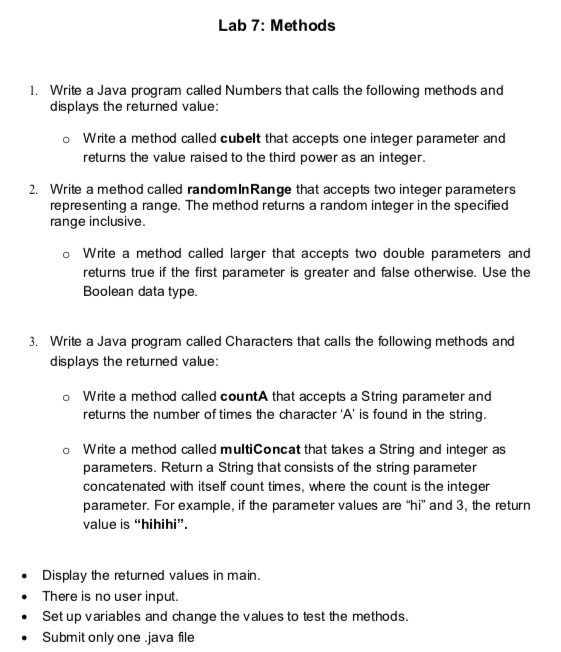I need java code for the following problem. Lab 7: Methods 1. Write a Java program called Numbers that calls the following methods and displays the returned value: Write a method called cubelt that accepts one integer parameter and returns the value raised to the third power as an integer. o Write a method called randominRange that accepts two integer parameters representing a range. The method returns a random integer in the specified range inclusive. 2. o Write a method...

Free Homework App